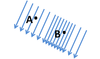# Newton, Units & Translation Flashcards Preview

## AP Physics > Newton, Units & Translation > Flashcards

Flashcards in Newton, Units & Translation Deck (70):
1

2

3

4

5

6

7

## What two characteristics are necessary to define a vector?

### Magnitude and direction.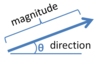8

9

## direction of a vector

### Direction provides spacial orientation, angle, or path. By convention, two perpendicular directions are fixed as positive (right and up) and their opposites as negative (left and down).10

## What is the magnitude of the velocity vector pointing directly down at 5 m/s?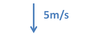11

## What is the direction of the velocity vector pointing directly up at 13 m/s?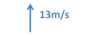12

## Describe the general process for vector addition.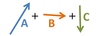### Vectors are added "tip to tail" so that there is a continuous pathway formed from the first arrow through the last one.  The sum vector is made by connecting the original tail to the final tip.13

## Describe the general process for vector subtraction.### Vectors are subtracted in the same process as addition: "tip to tail". The direction of the vector being subtracted must be reversed prior to adding.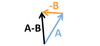14

## component vectors

### Component vectors represent the magnitude of a given vector along the x axis and y axis.  Generally these are referred to as, for instance, the "x component of [vector]". In the image below, vector A is shown with its x and y components as Ax and Ay respectively.15

## Give the formula for the x component of a force in the xy plane.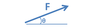### Fx = F * cos θ In general: [vector]x = [original vector] * cos θ16

## What is the x component of velocity for a ball thrown upwards at 4 m/s, 30º to the horizon? (sin(30º) = .5, cos(30º) = .86)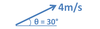17

## Give the formula for the y component of a force in the xy plane.### Fy = F * sin θ In general: [vector]y = [original vector] * sin θ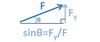18

## What is the y component of velocity for a ball thrown upwards at 4 m/s, 30º to the horizon? (sin(30º) = .5, cos(30º) = .86)19

20

21

22

23

24

25

26

27

28

29

30

31

32

33

34

35

36

37

38

39

40

41

42

43

44

45

46

47

48

49

## Normal force

### When two objects are touching, there exists an opposing force between the two objects and perpendicular to the surface in contact. This is the normal force. Often, on the AP exam, normal force is opposing the force due to gravity.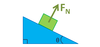50

51

52

53

54

55

56

57

## What formulas give the component forces for the force due to gravity on an inclined plane? (note that by covention the "x" axis is along the slope of the plane, and the "y" axis is perpendicular to the plane)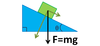### Fx=mg sinθ Fy=mg cosθ Since these are opposite from how we normally compute components, a way to remember the x component is: sine is for slope.58

## What calculation will give you the normal force on a box of mass m, on an inclined plane with angle θ?### FN = -mg cosθ Since the box is not sinking into the slope, nor launching up off of the slope, the net force acting perpendicular to the slope must be zero. Hence, Fy=-FN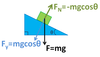59

## What is the force of static friction, if the box does not start to slide down the plane?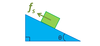### Fs = -mg sin(θ) There are two forces parallel to the slope of the plane; the component of gravity parallel to the plane, with magnitude mg sin(θ), and the force of static friction, pointing in the opposite direction. These forces must be equal and opposite to prevent motion.60

## In the pulley system demonstrated below, m2 is twice the mass of m1 and there is no net movement. What is the relationship between the tension in the string at points 1 and 2?### The tensions are equal. A key to all pulley questions is to remember that the tension in the string running through the system is always constant, since it is all one string.61

## What is the force F in the pulley apparatus below, with respect to the mass m1, assuming that all masses are stationary and no friction exists?### F = m1g There are two forces acting on m1; gravity and tension. If the box isn't moving, they must be equal and opposite, so T = -m1g. Since tension is constant through the string, the force on m2 from tension must also be F = -T = m1g.62

## What is the force F in the pulley apparatus below, with respect to the mass m2, assuming that all masses are stationary and no friction exists?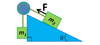### F = -m2g sinθ There are two forces acting on the box along the inclined plane: the x component of gravity and the tension in the string. If the box isn't moving, they must be equal and opposite. Hence F = T = -m2g sinθ.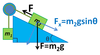63

64

65

66

67

68

69

## What is the direction of the force experienced by an object placed at point A in the gravitational field depicted below?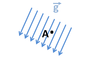### The object feels a force down and to the left. This is the direction the vectors in the vicinity of point A are pointing, and these vectors represet the forces experienced by objects at these locations. On the AP exam, fields are assumed to be of uniform density until told otherwise.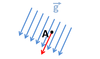70

## Where is the magnitude of the field depicted below greater, point A or point B?### The magnitude of the field is greater at point B. The magnitude of a field can be estimated from the density of the field lines in this location. The greater the density, the larger the magnitude of the field. The field lines are packed more tightly at point B than at point A, hence the magnitude of the field is greater there.